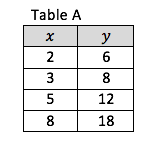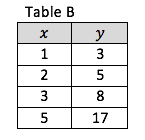# Functions

## Objective

Determine if functions are linear or nonlinear when represented as tables, graphs, and equations.

## Common Core Standards

### Core Standards

?

• 8.F.A.1 — Understand that a function is a rule that assigns to each input exactly one output. The graph of a function is the set of ordered pairs consisting of an input and the corresponding output. Function notation is not required in Grade 8.

• 8.F.A.3 — Interpret the equation y = mx + b as defining a linear function, whose graph is a straight line; give examples of functions that are not linear. For example, the function A = s² giving the area of a square as a function of its side length is not linear because its graph contains the points (1,1), (2,4) and (3,9), which are not on a straight line.

?

• 7.RP.A.2.B

## Criteria for Success

?

1. Determine if a function represented as a table or coordinate points is linear.
2. Determine if a function represented as an equation is linear.

#### Fishtank Plus

• Problem Set
• Student Handout Editor
• Vocabulary Package

## Anchor Problems

?

### Problem 1

The tables below show some input and output values for two different functions.
Determine if each table represents a linear or nonlinear function.### Problem 2

Determine which equations below represent linear functions. Be prepared to justify your reasoning.

Equation 1:   ${y = x^2 +1}$
Equation 2:   ${y=2x+1}$
Equation 3:   ${y={x \over 2}}$
Equation 4:   ${y=x^3}$

## Problem Set

?The following resources include problems and activities aligned to the objective of the lesson that can be used to create your own problem set.

?

### Problem 1

Which of the equations listed below are linear equations?

Equation 1:   ${C={2\pi}r}$
Equation 2:   ${A={\pi}r^2}$
Equation 3:   ${V={4\over3}{\pi}r^3}$

1. Equation 1 only
2. Equation 2 only
3. Equations 1 and 3
4. Equations 2 and 3

#### References

Question 7

From EngageNY.org of the New York State Education Department. New York State Testing Program Grade 8 Common Core Mathematics Test Released Questions May 2016. Internet. Available from https://www.engageny.org/resource/released-2016-3-8-ela-and-mathematics-state-test-questions/file/148616; accessed Oct. 27, 2017, 10:44 a.m..

### Problem 2

Complete the table so it represents a linear function.

 $x$ $y$ 2 0 4 5 18 7

?